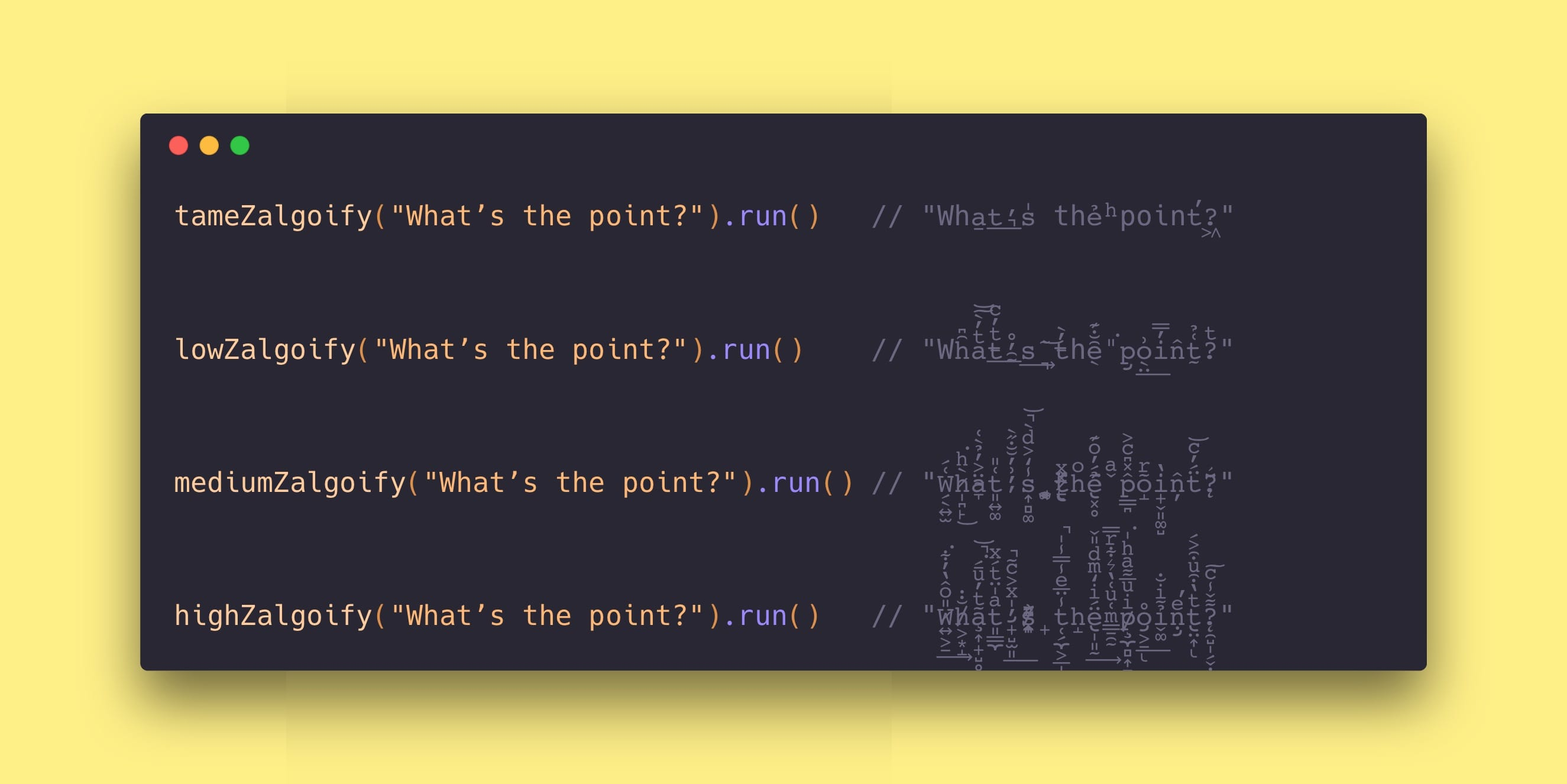A blog exploring functional programming and Swift.# Random Zalgo GeneratorLet’s create a random Zalgo text generator using the simple `Gen` type we defined in this week’s episode!

In this week’s episode we discussed the topic of “Composable Randomness”, which seeks to understand how randomness can be made more composable by using function composition. We also compared this with Swift 4.2’s new randomness API, which arguably is not composable, in the sense that it is not built from units that stand on their own and combine to form new units.

In the episode we built up some complex generators from simpler pieces, like a random array generator and a random password generator. In today’s Point-Free Pointer we want to walk you through another one: a random Zalgo text generator.

## Zalgo

Zalgo text is a style of text that introduces glitchy artifacts into the characters by inserting unicode combining characters, which build up and overlay on top of the characters you want to display. For example, P̨̀͞o̧͟in͡t̴-͠F͘re҉e͡. All of unicode’s combining characters are contained in the range 0x300 to 0x36F, and you can insert as many as you want into a string in order to glitch it up.

## The Gen type

The fundamental unit of randomness we explored was called the `Gen` type:

``````struct Gen<A> {
let run: () -> A
}``````

We showed that it has a `map`-like function, which behaves much like the `map` you know and love from arrays and optionals, and its precisely what allows you to build new generators out of old:

``````extension Gen {
func map<B>(_ f: @escaping (A) -> B) -> Gen<B> {
return .init { f(self.run()) }
}
}``````

## Some simple generators

It’s easy enough to cook up values of the `Gen` type. Often we can just wrap the new Swift 4.2 API in it:

``````func int(in range: ClosedRange<Int>) -> Gen<Int> {
return .init { Int.random(in: range) }
}

int(in: 0...10).run() // 3
int(in: 0...10).run() // 1
int(in: 0...10).run() // 7
int(in: 0...10).run() // 10``````

Here `int(in:)` is a function that takes a range and returns a generator of random numbers in that range, where we have just delegated the random calculation to `Int.random(in:)`.

However, there are some generators that the Swift APIs do not address at all, like a generator of randomly sized arrays with random elements:

``````extension Gen {
func array(count: Gen<Int>) -> Gen<[A]> {
return .init {
Array(repeating: (), count: count.run())
.map { self.run() }
}
}
}

int(in: 0...10).array(count: int(in: 0...3)).run() // [2, 7]
int(in: 0...10).array(count: int(in: 0...3)).run() // [6, 4, 3]
int(in: 0...10).array(count: int(in: 0...3)).run() // [8, 0]
int(in: 0...10).array(count: int(in: 0...3)).run() // []``````

So already `Gen` has given us a nice way to express something that does not exist in the Swift 4.2 API.

## Zalgo generator

Let’s start simple… can we make a generator for a random Zalgo character? We know the range that these characters live in, so its just a matter of choosing a random code point in that range and constructing a `String` from that value:

``````let zalgo = int(in: 0x300 ... 0x36f)
.map { String(UnicodeScalar(\$0)!) }

zalgo.run() // " ͉"
zalgo.run() // " ͚"
zalgo.run() // " ̊"
zalgo.run() // " ̓"``````

That was quite easy!

Next let’s create a generator of a random number of Zalgo characters together. We want this because the more Zalgo characters you use next to each other, the more intense the glitchiness is, e.g., P̅ö̔̇͆inͪt-F͛̑̓ͩrẽẻ versus P̡o҉̩̹̻̠ͅi͚̼͚̪ͅṋ̨t̘̹̯͚̭́-̡̗͉F̖́rẹ̛̖e̶̖̜̰̫͎.

``````func zalgos(intensity: Int) -> Gen<String> {
return zalgo
.array(count: int(in: 0...intensity))
.map { \$0.joined() }
}

let tameZalgos   = zalgos(intensity: 1)
let lowZalgos    = zalgos(intensity: 5)
let mediumZalgos = zalgos(intensity: 10)
let highZalgos   = zalgos(intensity: 20)

"a" + tameZalgos.run()   // ạ

"a" + lowZalgos.run()    // a͕̱̲ͫ

"a" + mediumZalgos.run() // a̢̯̟̓̽ͮͫ

"a" + highZalgos.run()   // ậ̵͇͚͍̗̿͌́͐̾̂͜͡``````

Here we were able to build the `zalgos(intensity:)` function by transforming the `zalgo` generator under the hood. The `intensity` determines the maximum number of combined Zalgo characters we are allowed to have.

Now that we have a way of building up many Zalgo characters of various intensities we can easily “Zalgo-ify” any string by simply interspersing Zalgo characters between the string’s characters:

``````func zalgoify(with zalgos: Gen<String>) -> (String) -> Gen<String> {
return { string in
return Gen {
string
.map { char in String(char) + zalgos.run() }
.joined()
}
}
}``````

We wrote this function in the “configuration first, data last” curried style. If you give it a Zalgo generator, it will give back a function that transforms any string into its Zalgo-ified version. Let’s use it!

``````let tameZalgoify   = zalgoify(with: tameZalgos)
let lowZalgoify    = zalgoify(with: lowZalgos)
let mediumZalgoify = zalgoify(with: mediumZalgos)
let highZalgoify   = zalgoify(with: highZalgos)

tameZalgoify("What’s the point?").run()   // "Wha̠t͟’͉s̍ thẻ ͪpoint̕?͖"

lowZalgoify("What’s the point?").run()    // "Wh̑͆aͭ̓̀͠͝t̵ͭ̓ͨ͟’̯̰̊s͢ ͉͏͂͝t̵̓̀hȇ̖̐͊ ̎͘p̡o̖̤͗͟i̓̿n̂t̰͑̉?ͭ"

mediumZalgoify("What’s the point?").run() // "W̗̖͍̫͑́h̷̩̪̙̀ͪ͘͜ä̴̞͐̓̉̀͑t͈͍͚͑̎’̦͗̓̆̐̋̀s͎̻͚̾̒͐ͩ̀̚͝ ̥̥̫͚̘ṯ̷̢ͯͯ͗́͘ͅhͦẻ̢͓̥́̓ͦ͊͊͘ ̌ͣp̳̪̂̽͆ͨ͐õ̝ͬi̟̬͈͚̺̔n̦̂ẗ́̓ͨ͝?̨̈́̌̄"

highZalgoify("What’s the point?").run()   // "W̷͍͕̱̎ͦ̂̔̓͋͘͢h̸͕͙̝̐̇a̧͎̟̺̥͖͂ͭ̓ͧ̄́͘̚͝t͈̳̼ͣ̍̈ͭ́ͯ’̡̟̺̫͈̍ͯ͐ͨ͂̚͟s̸͎̣̪̠̯͌ͬ͗͏̱̂ ̟t̜̗̼͕̲̩̪̗̦̾̈̅ͤ̾̿̾̍̚ͅh̝ë̢̩͈̰́ͥ̒ͫͩ̎̌͢ ̳̱̯̰ͫ͑ͧ͑̔͛͋ͬ̿p̸̧̼̻͎̱̺ͥͮ̅͌ͣͪ̍͘o̡͕̠̊͟ͅỉ̬͚͂ͥ̐ṇ̡ͤ̕t̢̤͎ͭ̔͒ͧ͒͐́ͅ?̨̯̺̩̗̬̣͌̌̾ͨ͠"``````

Wow! We’ve been able to progressively increase the Zalgo-ification of our string “What’s the point?”, and the entire generator was built from small, reusable units that work in isolation but also plug together in all types of interesting ways.

## Conclusion

This has been a fun demonstration of the power of composition. We started with a very simple type, `Gen<A>`, that had a `map` function, and a few generators (`int(in:)` and `array(count:)`), and from that we built a generator that can randomly Zalgo-ify any string. And it even comes with a dial to tune the intensity you want from your Zalgo-ification. This is the power of composition!

If this piques your interest, then you will probably be interested in this week’s episode “Composable Randomness”, where we go even deeper into this idea.

#### Subscribe to Point-Free

👋 Hey there! If you got this far, then you must have enjoyed this post. You may want to also check out Point-Free, a video series on functional programming and Swift.